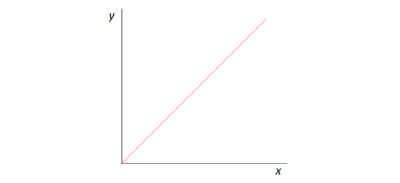# A Simple Introduction To The Principles Of Thermometry

As we navigate our day-to-day lives, we all get a feel for what temperature is. In fact, we even use common adjectives to describe temperature, such as hot, warm and cold. As such, we have a general intuition as to how a specific temperature differs from another. From a Physics standpoint, let’s start by answering some fundamental questions down below.

What is Temperature?

Temperature refers to the measure of the hotness or coolness of an object. In other words, it indicates the amount of thermal energy an object contains. The SI Unit to measure temperature is kelvin (K). Degree Celsius (ºC) is another commonly used metric.

What is a Temperature Scale?

A temperature scale is a calibrated system for measuring temperature, making it necessary for studying changes in temperature and heat transfer. A thermometer is an instrument that uses a temperature scale to measure temperature accurately.

Any physical property that changes with temperature is called a thermometric property. Hence, the substance used is called a thermometric substance. For instance, let’s take a look at the table below to identify some common thermometric properties and their uses in different types of thermometers:

 Thermometric Property Type of Thermometer 1. The volume of a fixed mass of liquid Liquid-in-glass thermometer, which either uses mercury or alcohol. 2. Electromotive force (e.m.f.) Thermocouple thermometer 3. The resistance of a piece of metal Resistive thermometers, also known as resistance temperature detectors (RTDs)

How to define a temperature scale?

In order to define a temperature scale, you’ll need the following:

1. A thermometric property that varies linearly with temperature. This means that the property changes by a fixed amount based on every unit change in temperature.

2. Two temperature points, also known as fixed points, which are standard degrees of hotness or coldness that are easy to obtain and is reproducible.Source: Wikimedia Commons (CC License)

In which y = physical property, and x = temperature, this graph depicts the linear relationship between a physical property and temperature.

Types of thermometer

Today, there are a variety of thermometers available. In this article, we’ll be covering the first two thermometers as listed in the table above.

1. Liquid-in-glass Thermometer

As one of the most common thermometers, you might be familiar with the liquid-in-glass thermometer. It works based on the principle of thermal expansion of substances. When the thermometer is subjected to heat, the liquid in the capillary (the slender glass tube) expands. Once the liquid is cooled, it’ll contract. Based on the resulting height of the liquid level, you’d be able to read off the temperature.

The liquid used has to meet a set of conditions:

• The liquid must not freeze at low temperatures
• The liquid should have a significantly high boiling point so that they won’t vaporise at high temperatures
• The liquid must be able to expand evenly with the temperature within the measuring range

Liquids that meet these properties are deemed suitable for use in liquid-in-glass thermometers, and are referred to as thermometric liquids.

Calibrating a liquid-in-glass thermometer:

The two fixed points used in a liquid-in-glass thermometer are:

• Steam point (upper fixed point): The temperature at which pure water boils at one atmospheric pressure of 100ºC.
• Ice point (lower fixed point): The temperature when pure ice melts at one atmospheric pressure of 0ºC.

Now that we’ve covered the factors to consider in choosing a thermometric substance and calibrating the two fixed points, the last two steps in calibrating a liquid-in-glass thermometer are as follows:

• Record down measurements of the respective physical property

Note down the values of the physical property of substance at the two fixed points.

• Set up the scale

Between the two fixed points, divide them equally into 100 parts. Each mark on the thermometer is a measure of 1ºC.

2. Thermocouple Thermometer

A thermocouple is formed by two types of wires which are made of different metals such as copper and iron. The two ends of both the wires meet to form two junctions.

The conditions for the thermocouple thermometer to work:

• Both junctions will each produce a small e.m.f., with a difference in temperature between them.
• The temperature can then be calculated using a millivoltmeter.
• One junction usually remains at a fixed temperature, while the other is used to measure the unknown temperature.
• The equation is: εαΔθ, where

ε = e.m.f. generated

Δθ = difference in temperature between both junctions

Conclusion

We’ve come to the end of our mini-lesson on the principles of thermometry and temperature. It may seem challenging to comprehend at first, especially the equations and calibration process. If you need further help in understanding these principles of thermometry and temperature, having the guidance of an experienced tutor might help! In that case, look no further! You’re always welcome to sign up for Physics Tuition to enhance your learning experience.